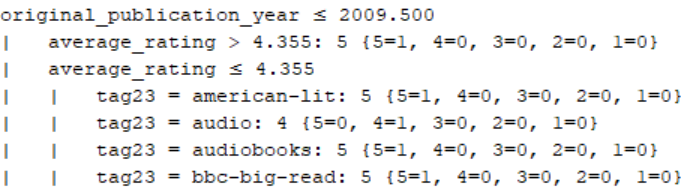# Decision tree -only one object in the leaf

Member Posts: 15Contributor I
edited November 2018 in Help

Hello,

I've heard that when I have only object assigned to a class in a leaf, it means that my tree is overtested.

Could you tell me if this is something wrong with my optimize operator?

`<?xml version="1.0" encoding="UTF-8"?><process version="8.1.003">  <context>    <input/>    <output/>    <macros/>  </context>  <operator activated="true" class="process" compatibility="8.1.003" expanded="true" name="Process">    <process expanded="true">      <operator activated="true" class="retrieve" compatibility="8.1.003" expanded="true" height="68" name="Retrieve bookratingsTagsUserNEW" width="90" x="179" y="85">        <parameter key="repository_entry" value="bookratingsTagsUserNEW"/>      </operator>      <operator activated="true" class="concurrency:optimize_parameters_grid" compatibility="8.1.003" expanded="true" height="124" name="Optimize Parameters (Grid)" width="90" x="447" y="85">        <list key="parameters">          <parameter key="Performance.accuracy" value="true,false"/>          <parameter key="Decision Tree.minimal_size_for_split" value="[0.0;0.0;0;linear]"/>          <parameter key="Decision Tree.confidence" value="[1.0E-7;0.5;10;linear]"/>        </list>        <process expanded="true">          <operator activated="true" class="split_data" compatibility="8.1.003" expanded="true" height="103" name="Split Data" width="90" x="45" y="238">            <enumeration key="partitions">              <parameter key="ratio" value="0.7"/>              <parameter key="ratio" value="0.3"/>            </enumeration>          </operator>          <operator activated="true" class="concurrency:parallel_decision_tree" compatibility="8.1.003" expanded="true" height="103" name="Decision Tree" width="90" x="179" y="85">            <parameter key="maximal_depth" value="100"/>            <parameter key="confidence" value="0.5"/>            <parameter key="minimal_leaf_size" value="1"/>            <parameter key="minimal_size_for_split" value="3"/>          </operator>          <operator activated="true" class="apply_model" compatibility="8.1.003" expanded="true" height="82" name="Apply Model" width="90" x="246" y="238">            <list key="application_parameters"/>          </operator>          <operator activated="true" class="performance_classification" compatibility="8.1.003" expanded="true" height="82" name="Performance" width="90" x="380" y="187">            <parameter key="main_criterion" value="accuracy"/>            <list key="class_weights"/>          </operator>          <connect from_port="input 1" to_op="Split Data" to_port="example set"/>          <connect from_op="Split Data" from_port="partition 1" to_op="Decision Tree" to_port="training set"/>          <connect from_op="Split Data" from_port="partition 2" to_op="Apply Model" to_port="unlabelled data"/>          <connect from_op="Decision Tree" from_port="model" to_op="Apply Model" to_port="model"/>          <connect from_op="Apply Model" from_port="labelled data" to_op="Performance" to_port="labelled data"/>          <connect from_op="Apply Model" from_port="model" to_port="model"/>          <connect from_op="Performance" from_port="performance" to_port="performance"/>          <portSpacing port="source_input 1" spacing="0"/>          <portSpacing port="source_input 2" spacing="0"/>          <portSpacing port="sink_performance" spacing="0"/>          <portSpacing port="sink_model" spacing="0"/>          <portSpacing port="sink_output 1" spacing="0"/>        </process>      </operator>      <connect from_op="Retrieve bookratingsTagsUserNEW" from_port="output" to_op="Optimize Parameters (Grid)" to_port="input 1"/>      <connect from_op="Optimize Parameters (Grid)" from_port="performance" to_port="result 2"/>      <connect from_op="Optimize Parameters (Grid)" from_port="model" to_port="result 1"/>      <portSpacing port="source_input 1" spacing="0"/>      <portSpacing port="sink_result 1" spacing="0"/>      <portSpacing port="sink_result 2" spacing="0"/>      <portSpacing port="sink_result 3" spacing="0"/>    </process>  </operator></process>`

For example something like that, where to the classses  I have one object assigned:• Moderator, RapidMiner Certified Analyst, Member, University Professor Posts: 568Unicorn
Solution Accepted

First, let's see: overfitted means that your model learned too much of your data and if you present it with new data, your model might throw strange, undesired results. On the other hand, underfitted means that your model is too simple, it won't be able to look at patterns. I have never heard of overtested, (testing extensively is always desirable).

To prevent overfitting, you should use pre-pruning first, then pruning, as we discussed earlier this week or last week. If you are already pre-pruning and pruning the tree, my next guess would be that the proportions you are using to train your model and test it aren't in good shape, but you are using 0.7 and 0.3, which is safe, which left me wondering it might be one of two things:

1. The amount of data you are using to train your decision tree is too small.
2. The configuration of the "Optimize Parameters (Grid)" process is less than ideal.

If it's the first one, it depends on the data you have, and we don't know how your data looks like (it's not part of the XML). If it's the second one, it depends on what kind of parameter you want to optimize.

Taking a look at the configuration of the Optimize Parameters (Grid) operator, I see you chose to optimize the "accuracy" parameter from the "Performance" parameter, and that is a "nope! wrong answer!". Let's put it that way: if your car runs too slow, what fix would you apply? Use another speedometer to measure in km/h instead of mph, or put a newer, better engine in your car? This is the same: You don't want to optimize the way you measure the performance of the algorithm (your speedometer), you want to optimize your algorithm (your engine). An educated guess would be something like this for a decision tree:Notice that I selected some of the parameters from the Decision Tree, that's the part I want to optimize.

Notice that the more parameters you add to your "Selected Parameters" list, the more combinations are tested with your split data, the more RAM you need and the more processing power will be used, so be vigilant of that number below to pick your favorite settings.

As for proper credits, @Thomas_Ott is the man with a plan. His video might shed some light on how to do proper parameter optimization: https://www.youtube.com/watch?v=R5vPrTLMzng

Hope this helps.